18

# Graphs of the trigonometric functions

Zeros of a function

The period of a function

The graph of y = sin x

The graph of y = cos x

The graph of y = sin ax

The graph of y = tan x

LET US BEGINwith some algebraic language.  When we write "nπ," where n could be any integer, we mean "any multiple of π."

0,  ±π,  ±2π,  ±3π, .  .  .

Zeros

By the zeros of sin θ we mean those values of θ for which sin θ will equal 0.

Now, where are the zeros of sin θ?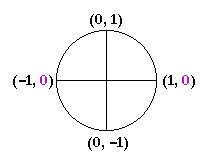We saw in Topic 15 on the unit circle that the value of sin θ is equal to the y-coordinate.  Hence, sin θ = 0 at θ = 0 and θ = π -- and at all angles coterminal with them.  In other words,

(1) .  .  .  .  .  .  .  .   . sin θ = 0  when  θ = nπThey will be the x-intercepts of the graph of the sine.

Line (1) will be true, moreover, for any argument θ.  For example,

sin 2x = 0  when 2x = nπ;

that is, when

 x = nπ 2 .
 Which numbers are these?  The multiples of π2 :
 0,  ± π2 ,  ±π,  ± 3π 2 , . . .

Problem 1.   Where are the zeros of  y =sin 3x?

At 3x = nπ; that is, at

 x = nπ 3 .

Which numbers are these?

 The multiples of π3 .

The period of a function

When the values of a function regularly repeat themselves, we say that the function is periodic.  The values of  sin θ  regularly repeat themselves every 2π units.sin (θ + 2π) = sin θ.

sin θ therefore is periodic.  Its period is 2π.  (See the previous topic, Line values.)

Definition.  If, for all values of x, the value of a function at x + p
is equal to the value at x --

If  f(x + p) = f(x)

-- then we say that the function is periodic and has period p.

The graph of y = sin x

The zeros of y = sin x are at the multiples of π.  It is there that the graph crosses the x-axis, because there y = 0.  And its period is 2π. But what is the maximum value of the graph and what is its minimum value?sin x has a maximum value of 1 at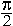, and a minimum of −1 at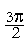—and at all angles coterminal with them.

Here is the graph of y = sin x:The independent variable x is the radian measure.  x may be any real number.We may imagine the unit circle rolled out, in both directions, along the x-axis.  (See Topic 15:  The unit circle.)

Problem 2. Vocabulary.

a)  In the function y = sin x, what is its domain?< x <.

b)  What is the range of y = sin x?

−1 ≤ sin x ≤ 1.

The graph of y = cos xThe graph of y = cos x is the graph of y = sin x  translatedunits to the left.  It is sin (x +).  And sin (x +)  =  cos x.  The student familiar with the sum formula can easily prove that. (Topic 20.)

On the other hand, it is possible to see directly that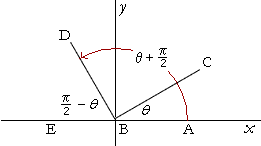Topic 16.  Angle CBD is a right angle.

The graph of y = sin ax

Since the graph of  y = sin x  has period 2π, then the constant a in

y = sin ax

indicates the number of periods in an interval of length 2π.  (In y = sin x, a = 1.)

For example, if a = 2 --

y = sin 2x

-- that means there are 2 periods in an interval of length 2π.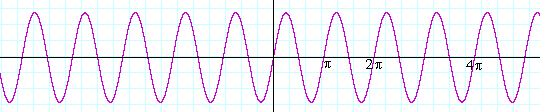If a = 3 --

y = sin 3x

-- there are 3 periods in that interval: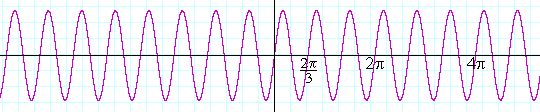While if a = ½ --

y = sin ½x

-- there is only half a period in that interval:The constant a thus signifies how frequently the function oscillates; so many radians per unit of x.

(When the independent variable is the time t, as it often is in physics, then the constant is written as ω ("omega"): sin ωt.  ω is called the angular frequency; so many radians per second.)

Problem 3.

a)   For which values of x are the zeros of y = sin mx?

 At mx = nπ; that is, at x = nπ m .

b)   What is the period of y = sin mx?

 2π m .  Since there are m periods in 2π, then one period

is 2π divided by m.

Compare the graphs above.

Problem 4.   y = sin 2x.

a)   What does the 2 indicate?

In an interval of length 2π, there are 2 periods.

b)   What is the period of that function?

 2π 2 = π

c)  Where are its zeros?

 At x = nπ 2 .

Problem 5.   y = sin 6x.

a)   What does the 6 indicate?

In an interval of length 2π, there are 6 periods.

b)   What is the period of that function?

 2π  6 = π3

c)  Where are its zeros?

 At x = nπ 6 .

Problem 6.   y = sin ¼x.

a)   What does ¼ indicate?

In an interval of length 2π, there is one fourth of a period.

b)   What is the period of that function?

2π/¼ = 2π· 4 = 8π.

c)  Where are its zeros?

 At x = nπ ¼ =  4nπ.

The graph of y = tan x

Here is one period of the graph of y = tan x:Why is that the graph?  It has effectively been explained in the previous topic, where we considered the line value DE of tan x in quadrants IV and I.In quadrant IV, tan x -- the line value DE -- is negative and takes on all possible negative values:

−∞ < tan x < 0.

At x = 0, tan x = 0.

And finally in quadrant I, tan x takes on all positive values:

0 < tan x < ∞.

And so in the interval from −to, tan x takes on all its possible values. That interval constitutes a complete period of y = tan x.

Here again is the graph.At the quadrantal angles  −and, tan x has no value. Therefore the lines x = −and x =are vertical asymptotes.

Here is the complete graph of  y = tan x.The graph of Quadrants IV and I is repeated in Quadrant II (where tan x is negative) and quadrant III (where tan x is positive), and periodically along the entire x-axis.

Problem 7. What is the period of y = tan x?

 One period is from − π2 to π2 . Hence the period is the

distance between those two points: π.

Next Topic:  Inverse trigonometric functions